# 机器学习多步时间序列预测解决方案

## 1 简介

• 多步递归预测（recursive multi-step forecasting）
• 多步直接预测（direct multi-step forecasting）
• 直接&递归融合预测（direct-recursive hybrid forecasting）
• 多输出预测（multiple output forecasting）

``git clone https://github.com/aws-samples/multi-step-forecasting-blog-sample-code``

## 2 多步递归预测（recursive multi-step forecasting）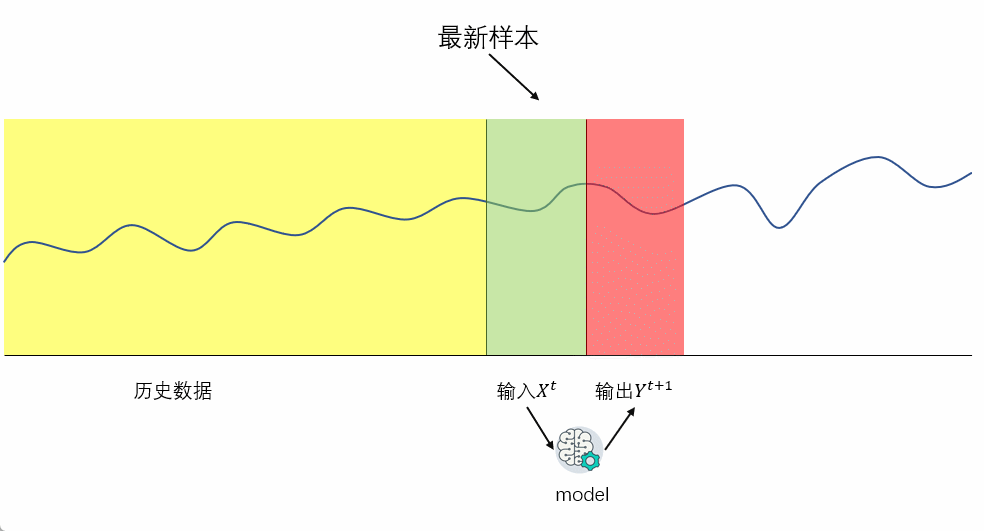• 需要的模型数量固定
• 计算量较低
• 预测的偏差（bias）相对于多步直接预测（后面会讲到）较低

• 由于使用上一步的预测值作为输入，预测误差会随着时间传递扩大，预测的方差（variance）较高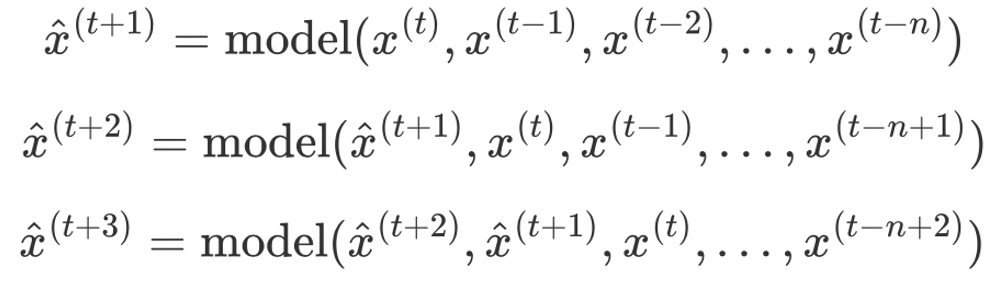1. rgnp : 预测目标，实际 GNP(gross national product，国民生产总值)
2. pgnp : 潜在GNP
3. ulc : 单位劳动成本
4. gdfco : 不包括食物和能源在内的个人消费支出的固定权重平减指数
5. gdf : 固定重量 GNP 缩减指数
6. gdfim : 定量进口减缩指数
7. gdfcf : 个人消费支出中食品的固定重量平减指数
8. gdfce : 个人消费支出中能量的固定权重平减指数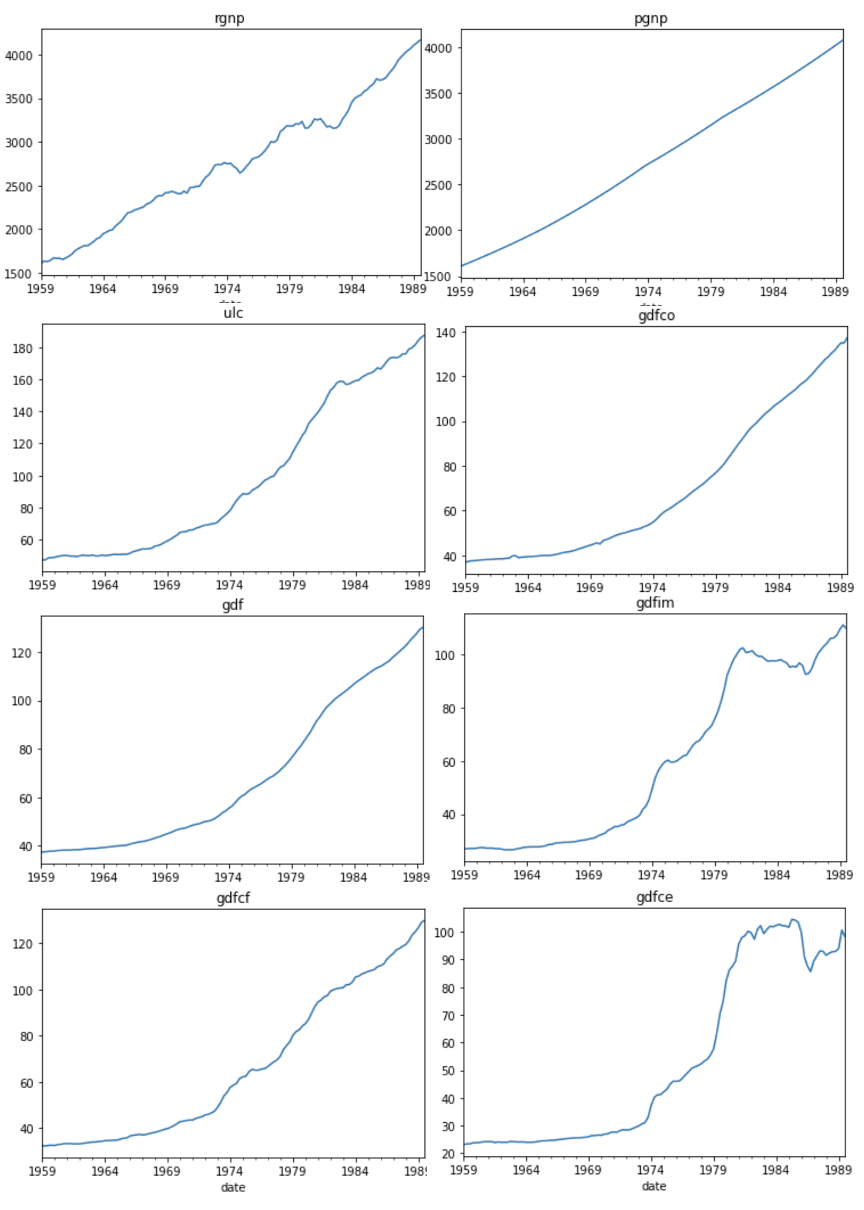``! pip install lightgbm``

``````import pandas as pd
import numpy as np
import lightgbm as lgb
from sklearn.metrics import mean_squared_error
from sklearn.multioutput import MultiOutputRegressor

# 将date作为索引
df['date'] = pd.to_datetime(df['date'])
df.index = df['date']
df.drop('date', axis=1, inplace=True)

target = 'rgnp'

X_train = df[: int(len(df) * 0.8)]
y_train = df[: int(len(df) * 0.8)]

X_test = df[int(len(df) * 0.8) :]
y_test = df[int(len(df) * 0.8) :]
``````

MultiOutputRegressor使用相同的feature来预测多个目标，通过该对象，可以针对每个目标训练出一个回归器。

``model = MultiOutputRegressor(lgb.LGBMRegressor(objective='regression')).fit(X_train, y_train)``

``````results = []
data = X_test
for i in range(12):
data = pd.DataFrame(model.predict(data), columns=data.columns, index=data.index)
results.append(data)

# make future targets
for i in range(12):
df['rgnp_{}'.format(i)] = df['rgnp'].shift(-i)

df.dropna(inplace=True)
df.drop('rgnp', axis=1, inplace=True)

targets = [item for item in df.columns if 'rgnp' in item]

X_train = df.drop(targets, axis=1)[: int(len(df) * 0.8)]
y_train = df[targets][: int(len(df) * 0.8)]

X_test = df.drop(targets, axis=1)[int(len(df) * 0.8) :]
y_test = df[targets][int(len(df) * 0.8) :]

(y_test - pred).abs().mean()``````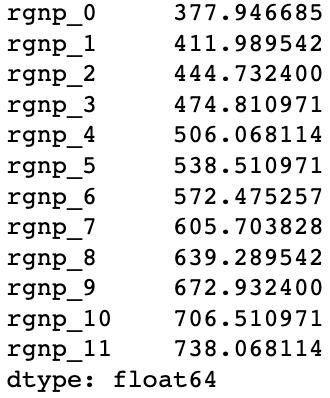## 3 多步直接预测（direct multi-step forecasting）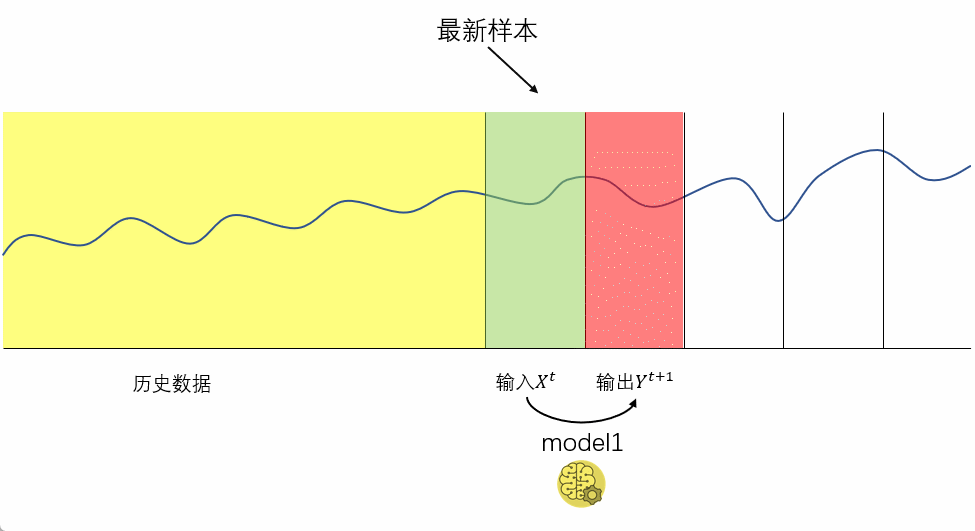• 与递归预测相比，由于不会误差传递，预测方差（variance）更低

• 预测较多时间步时，计算效率低（需要预测多少时间步，就需要训练多少个模型）
• 与递归预测相比，预测偏差（bias）较高，因为较远的目标无法获取与其相近的数据。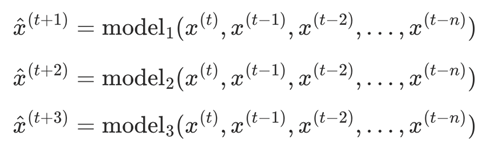``````import pandas as pd
import numpy as np
import lightgbm as lgb
from sklearn.metrics import mean_squared_error
from sklearn.multioutput import MultiOutputRegressor

df['date'] = pd.to_datetime(df['date'])
df.index = df['date']
df.drop('date', axis=1, inplace=True)

# make future targets
for i in range(12):
df['rgnp_{}'.format(i)] = df['rgnp'].shift(-i-1)

df.dropna(inplace=True)
``````

``````targets = [item for item in df.columns if 'rgnp_' in item]

X_train = df.drop(targets, axis=1)[: int(len(df) * 0.8)]
y_train = df[targets][: int(len(df) * 0.8)]

X_test = df.drop(targets, axis=1)[int(len(df) * 0.8) :]
y_test = df[targets][int(len(df) * 0.8) :]

model = MultiOutputRegressor(lgb.LGBMRegressor(objective='regression')).fit(X_train, y_train)
``````

``````targets = [item for item in df.columns if 'rgnp_' in item]

X_train = df.drop(targets, axis=1)[: int(len(df) * 0.8)]
y_train = df[targets][: int(len(df) * 0.8)]

X_test = df.drop(targets, axis=1)[int(len(df) * 0.8) :]
y_test = df[targets][int(len(df) * 0.8) :]

model = MultiOutputRegressor(lgb.LGBMRegressor(objective='regression')).fit(X_train, y_train)
``````

``````pred = pd.DataFrame(model.predict(X_test), columns=targets)
pred.index = y_test.index
(y_test - pred).abs().mean()
``````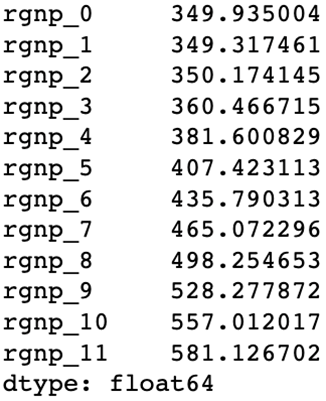## 4 多步直接&递归融合预测（direct-recursive hybrid forecasting）

1. 创建递归模型
2. 创建直接预测模型，但预测模型的输入还包括递归模型在先前时间步中所做的预测作为输入值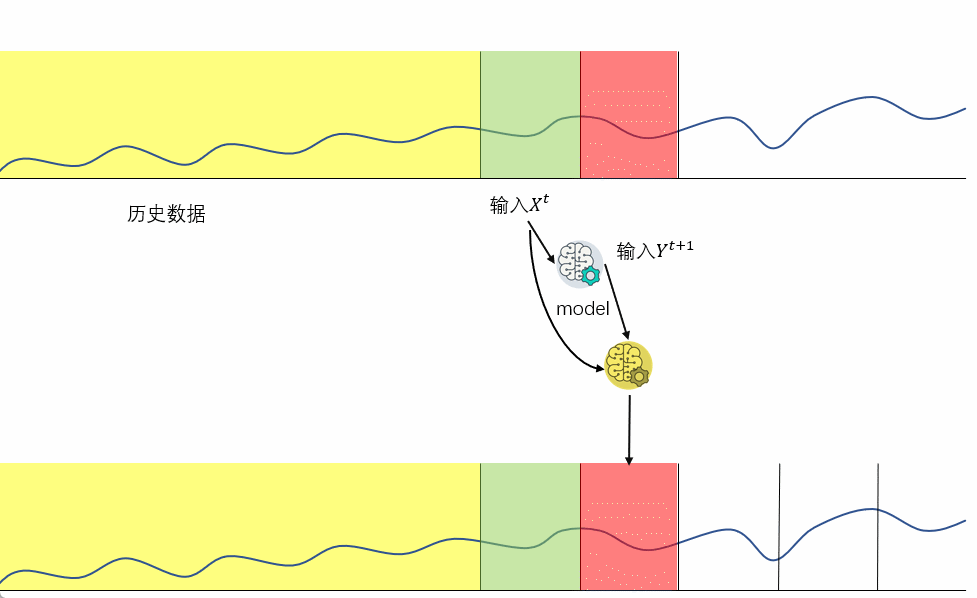• 结合直接预测来在一定程度上避免误差传播，降低了预测方差（variance）
• 结合递归预测，拥有较低的预测偏差（bias）

• 计算量比前面两种方法更大
• 实现复杂度较高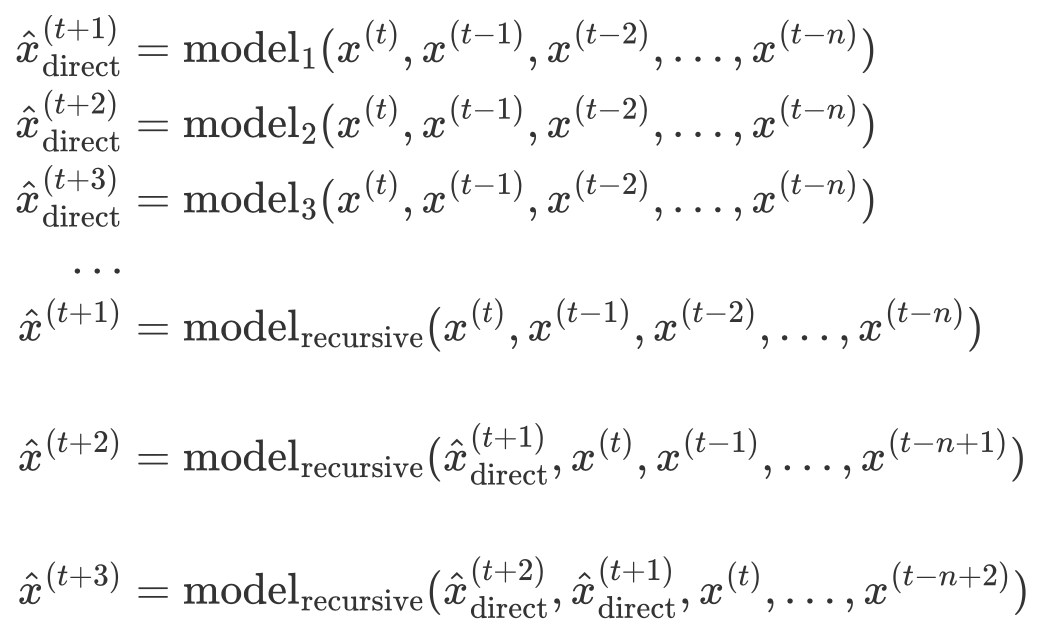``````import pandas as pd
import numpy as np
import lightgbm as lgb
from sklearn.metrics import mean_squared_error
from sklearn.multioutput import MultiOutputRegressor

df['date'] = pd.to_datetime(df['date'])
df.index = df['date']
df.drop('date', axis=1, inplace=True)
``````

``````target = 'rgnp'

X_train = df[: int(len(df) * 0.8)].iloc[:-1]
y_train = df[: int(len(df) * 0.8)].shift(-1).dropna()

X_test = df[int(len(df) * 0.8) :].iloc[:-1]
y_test = df[int(len(df) * 0.8) :].shift(-1).dropna()

model = MultiOutputRegressor(lgb.LGBMRegressor(objective='regression')).fit(X_train, y_train)
``````

``````results = []
data = X_test
for i in range(12):
data = pd.DataFrame(model.predict(data), columns=data.columns, index=data.index)
results.append(data)``````

``````# make future targets
for i in range(12):
df['rgnp_{}'.format(i+1)] = df['rgnp'].shift(-i-1)

df.dropna(inplace=True)

targets = [item for item in df.columns if 'rgnp_' in item]

X_train = df.drop(targets, axis=1)[: int(len(df) * 0.8)]
y_train = df[targets][: int(len(df) * 0.8)]

X_test = df.drop(targets, axis=1)[int(len(df) * 0.8) :]
y_test = df[targets][int(len(df) * 0.8) :]``````

``````models = []
for i in range(1, 13):

model = lgb.LGBMRegressor(objective='regression').fit(X_train, y_train['rgnp_{}'.format(i)])
X_train['rgnp_{}'.format(i)] = y_train['rgnp_{}'.format(i)]
models.append(model)``````

``````final_results = []

for i in range(12):
final_results.append(models[i].predict(X_test))
X_test['rgnp_{}'.format(i + 1)] = results[i]['rgnp']
``````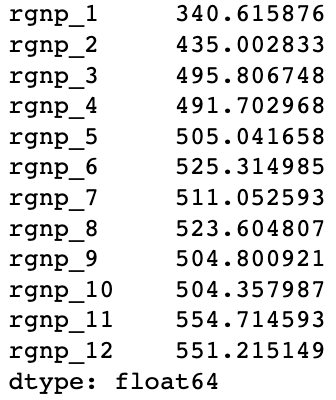## 5 多输出预测（multiple output forecasting）

• 不会有误差传递风险
• 同时学习多个输出，能够找到每个时间步的关联
• 由于神经网络的特殊性，对特征工程能力的要求较低

• 计算量过大
• 只能使用神经网络，不能使用tree-based模型
• 会有过拟合的风险，需要较多数据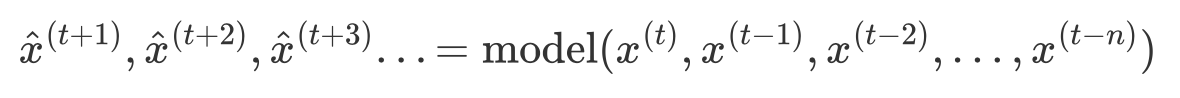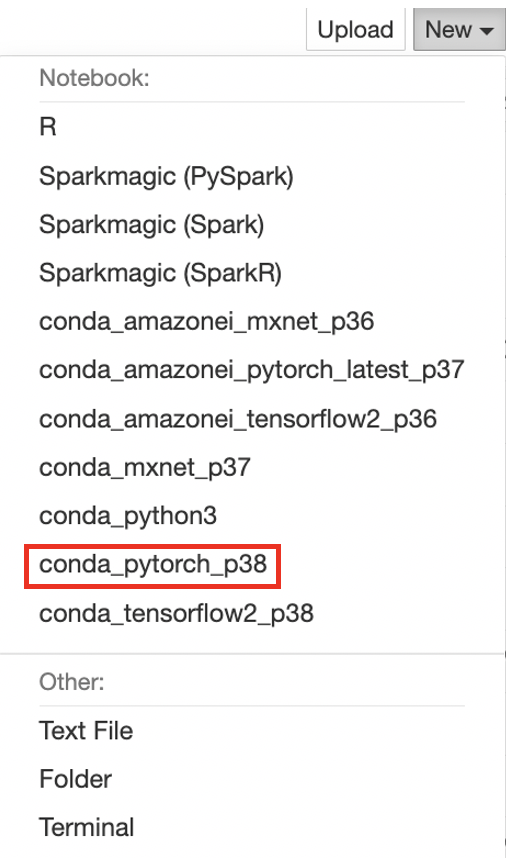``````import pandas as pd

import torch
import torch.nn as nn
import torch.optim as optim

import pandas as pd
import numpy as np
import lightgbm as lgb
from sklearn.metrics import mean_squared_error

df['date'] = pd.to_datetime(df['date'])
df.index = df['date']
df.drop('date', axis=1, inplace=True)

# make future targets
for i in range(12):
df['rgnp_{}'.format(i)] = df['rgnp'].shift(-i-1)

df.dropna(inplace=True)

targets = [item for item in df.columns if 'rgnp_' in item]

X_train = df.drop(targets, axis=1)[: int(len(df) * 0.8)]
y_train = df[targets][: int(len(df) * 0.8)]

X_test = df.drop(targets, axis=1)[int(len(df) * 0.8) :]
y_test = df[targets][int(len(df) * 0.8) :]
``````

``````class Raotbl6Dataset(Dataset):
"""Face Landmarks dataset."""

def __init__(self, X_train, y_train):
self.X_train = X_train
self.y_train = y_train

def __len__(self):
return len(self.X_train)

def __getitem__(self, idx):

if torch.is_tensor(idx):
idx = idx.tolist()

X = torch.Tensor(self.X_train.iloc[idx].values)
y = torch.Tensor(self.y_train.iloc[idx].values)
return X, y
``````

``````class Model(nn.Module):
def __init__(self, in_feats, out_feats=12, hidden_units=32):
super(Model, self).__init__()

self.net = nn.Sequential(
nn.Linear(in_feats, hidden_units),
nn.ReLU(),
nn.Linear(hidden_units, out_feats)
)

def forward(self, x):
return self.net(x)``````

``````NUM_EPOCHS = 500
LEARNING_RATE = 2e-4
device = 'cuda' if torch.cuda.is_available() else 'cpu'

dataset = Raotbl6Dataset(X_train, y_train)
criterion = torch.nn. MSELoss()

model = Model(8).to(device)
opt = optim.Adam(model.parameters(), lr=LEARNING_RATE, betas=(0.5, 0.999))

for epoch in range(NUM_EPOCHS):
model.train()
for batch_idx, (X, y) in enumerate(dataloader):

X, y = X.to(device), y.to(device)
pred = model(X)
loss = criterion(y, pred)

loss.backward()
opt.step()
print(
f"Epoch [{epoch}/{NUM_EPOCHS}] \
ERROR: {loss:.4f}"
)

pred = pd.DataFrame(model(torch.Tensor(X_test.values).to(device)), columns=y_test.columns, index=y_test.index
(y_test - pred).abs().mean()
``````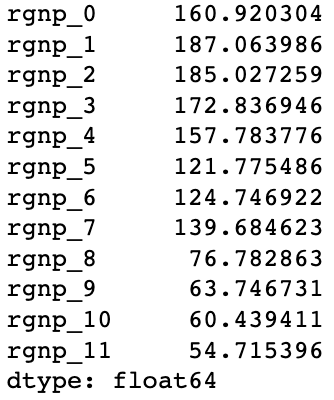## 6 总结

 算法 优点 缺点 多步递归预测 •需要的模型数量固定 • 计算量较低 • 预测的偏差（bias）相对于多步直接预测（后面会讲到）较低 •由于使用上一步的预测值作为输入，预测误差会随着时间传递扩大，预测的方差（variance）较高 多步直接预测 • 与递归预测相比，由于不会误差传递，预测方差（variance）更低 • 预测较多时间步时，计算效率低（需要预测多少时间步，就需要训练多少个模型） • 与递归预测相比，预测偏差（bias）较高，因为较远的目标无法获取与其相近的数据。 多步直接&递归融合预测 • 结合直接预测来在一定程度上避免误差传播，降低了预测方差（variance） • 结合递归预测，拥有较低的预测偏差（bias） • 计算量比前面两种方法更大 • 实现复杂度较高 多输出预测 • 无误差传递风险 • 同时学习多个输出，能够找到每个时间步的关联 • 由于神经网络的特殊性，对特征工程能力的要求较低 • 计算量过大 • 只能使用神经网络，不能使用tree-based模型 • 会有过拟合的风险，需要较多数据

## 本篇作者### 刘明### 陈恒智